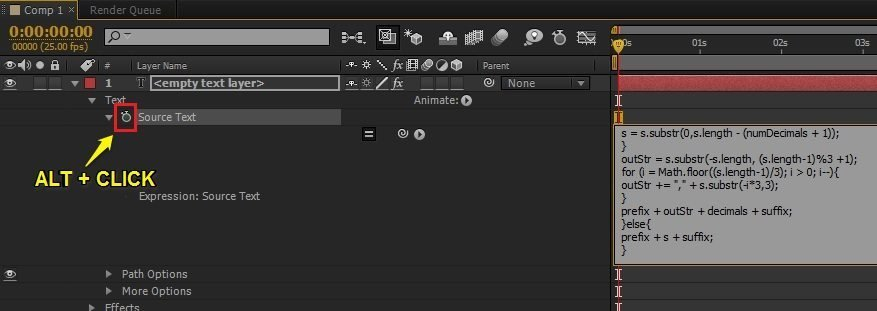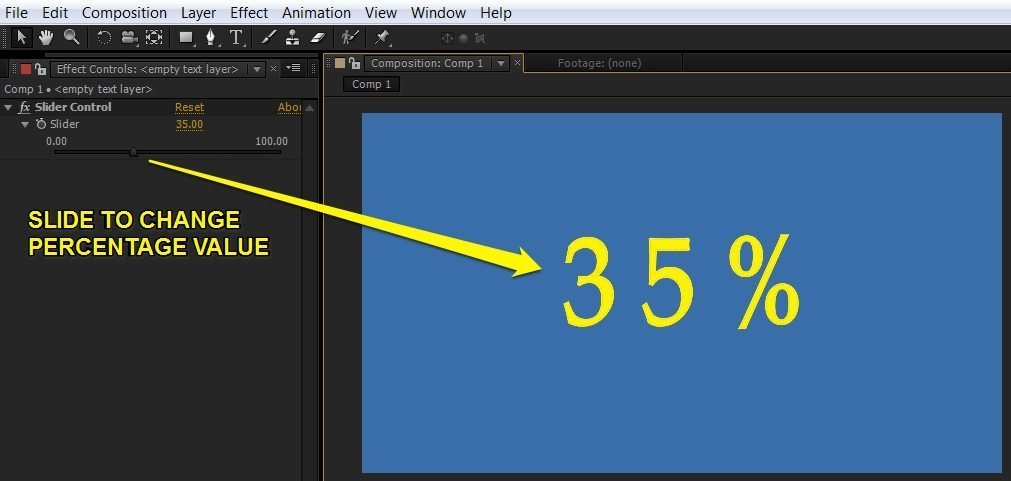After Effects Scripts and Expressions allow us to automate things and achieve effects without much hassle. With few lines of expressions, we can very easily create a percentage counter in After Effects.

In this tutorial, we will create a percentage counter that goes from 1% to 100%. There is no restriction in minimum and maximum values so you can also start and end with any random numbers.

To create a percentage counter, first you need to understand what expressions are and how to use them. This tutorial on ‘How to apply expressions‘ might help you out.

Once you have a clear understanding of expressions. Open up After Effects and create a new composition. But, before you proceed, copy the expression below and save it in a text file or notepad.

```slider = effect("Slider Control")("Slider");
numDecimals = 0;
commas = false;
dollarSign = false;
percentSign = true;
s = slider.value.toFixed(numDecimals);
prefix = "";
suffix = "";
if (s == "-"){
prefix = "-";
s = s.substr(1);
}
if(dollarSign) prefix += "\$";
if(percentSign) suffix = "%";
if (commas){
decimals = "";
if (numDecimals > 0){
decimals = s.substr(-(numDecimals + 1));
s = s.substr(0,s.length - (numDecimals + 1));
}
outStr = s.substr(-s.length, (s.length-1)%3 +1);
for (i = Math.floor((s.length-1)/3); i > 0; i--){
outStr += "," + s.substr(-i*3,3);
}
prefix + outStr + decimals + suffix;
}else{
prefix + s + suffix;
}```

After you save the expression,

1. Go to LAYER > NEW > TEXT to create a new text layer. At this point, you can type anything or simply leave it blank.
2. Now we need to create a slider, it will help in controlling and animated our percentage counter. Select the text layer, then go into EFFECTS > EXPRESSION CONTROLS > SLIDER CONTROL.
3. It’s time to set the range of our slider. To do that, go to the effects panel and right click on the slider, just underneath where it says SLIDER CONTROL, then click on EDIT VALUE.4. Set the slider range minimum value to 0 and maximum value to 100. For this tutorial, I am using 0 and 100 since these are the most common and universally accepted values for percentage, but you can also use any random numbers.5. Now, all we are left with is applying the expression to our text layer. To do that, expand the TEXT LAYER by clicking on the small arrow icon before the color label, then expand the TEXT to reveal the SOURCE TEXT, as shown in the image below.6. Hold down ALT on your keyboard and click on the SOURCE TEXT stopwatch to open the expressions box. Delete any existing codes and paste the new expression (One that you saved in the text file/notepad).7. Click anywhere, but out of the expressions box to save it. Once it is saved, use the slider to increase and decrease the percentage value.Though I have tried my best to explain all the steps very clearly, Still if you face any difficulty, download my project file for reference.